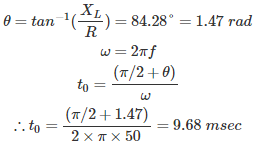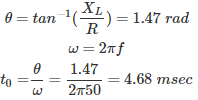# MCQs on Power Systems

##### Page 30 of 67. Go to page 1 2 3 4 5 6 7 8 9 10 11 12 13 14 15 16 17 18 19 20 21 22 23 24 25 26 27 28 29 30 31 32 33 34 35 36 37 38 39 40 41 42 43 44 45 46 47 48 49 50 51 52 53 54 55 56 57 58 59 60 61 62 63 64 65 66 67
01․ Three generators rated 100 MVA, 11 kV have an impedance of 0.15 pu each. If in the same plant, these generators are being replaced by a single equivalent generator, the effective impedance of equivalent generator will be
0.15 pu
0.45 pu
0.05 pu
0.25 pu

Given impedance value = 0.15 pu When three generators are replaced by a single generator, their impedance are connected parallelly. So, their equivalent impedance will be one-third of each impedance. New impedance = 0.15/3 = 0.05 pu

02․ Making current is a------------ value?
average
RMS
peak value
any of the above

The instantaneous maximum or peak value of the current flowing through the circuit breaker at the instant of closing of circuit breaker is called making current. Making current = 2.55 × Breaking current

03․ Breaking current is a ------------------ value?
RMS
average
maximum
any of the above

The RMS value of short circuit fault current passing through the circuit breaker at the time of opening of circuit breaker is called breaking current.

04․ If the fault is very nearer to the generator, the fault current is
less
larger
zero
any of the above

If the fault is very nearer to the substation or generator, reactance of the network is small. So that fault current is larger. Fault current If ∝ 1/X Where X = Reactance of the network Therefore, compare to single transmission line, parallel line or double line has low reactance. So that the fault current is more in case of parallel lines.

05․ A transmission line has impedance of (0.005 + j0.05) pu. Find the line at which maximum value of negative DC off set current is produced, if it is applied with Vm sinωt?
4.68 msec
9.68 msec
1.47 msec
2.63 msec

The time at which maximum value of negative DC off set current produced is t0, when it is applied with Vm sinωt. t0 = (π/2 + θ)/ω06․ A transmission line has impedance of (0.005 + j0.05) pu. Find the line at which maximum value of negative DC off set current is produced, if it is applied with Vm cosωt?
4.68 msec
9.68
1.47 msec
2.63 msec

The time at which maximum value of negative DC off set current produced is t0, when it is applied with Vm cosωt. t0 = θ/ω Where,07․ Find the relation between sub transient (X"), transient (X') and synchronous reactance (X) ?
X" > X' >X
X" < X' > X
X" > X' < X
X" < X' < X

The relation between sub transient (X"), transient (X') and synchronous reactance (X) is X" < X' < X Therefore, the relation between sub transient current, transient current, steady state current is, sub transient current > transient current > steady state current.

08․ A generator has reactances of sub transient (X")= 0.5 pu, transient (X') = 0.8 pu and synchronous reactance (X) = 5 pu. The voltages at these reactances are 1.2 V, 1.1 V and 1 V respectively. Find the corresponding sub transient fault current in pu?
1.375 pu
0.2 pu
2.4 pu
3.975 pu

Sub transient fault current = E"/X" Where, X" = Sub transient reactance E" = The voltage at sub transient reactance Sub transient fault current = 1.2/0.5 = 2.4 pu

09․ When series reactor is used in generating stations, the fault current magnitude is
increased
reduced
remains unaffected
any of the above

Fault current If ∝ 1/X Where X = Reactance of the network Therefore, with series reactor, the fault current magnitude is reduced. It is preferred in the generating stations in some special applications for limiting the current flowing through the low MVA rating circuit breakers.

10․ Operator "a" rotates a vector
clock wise direction
anti clock wise direction
either 1 or 2
nether 1 nor 2

For reducing the number of vectors and to get the relation between the vectors operator "a" is used. Operator "a" rotates a vector in anti clock wise direction. a = 1∠ 120° a² = 1∠ 240° = 1∠ -120° a³ = 1∠ 360° = 1

<<<2829303132>>>